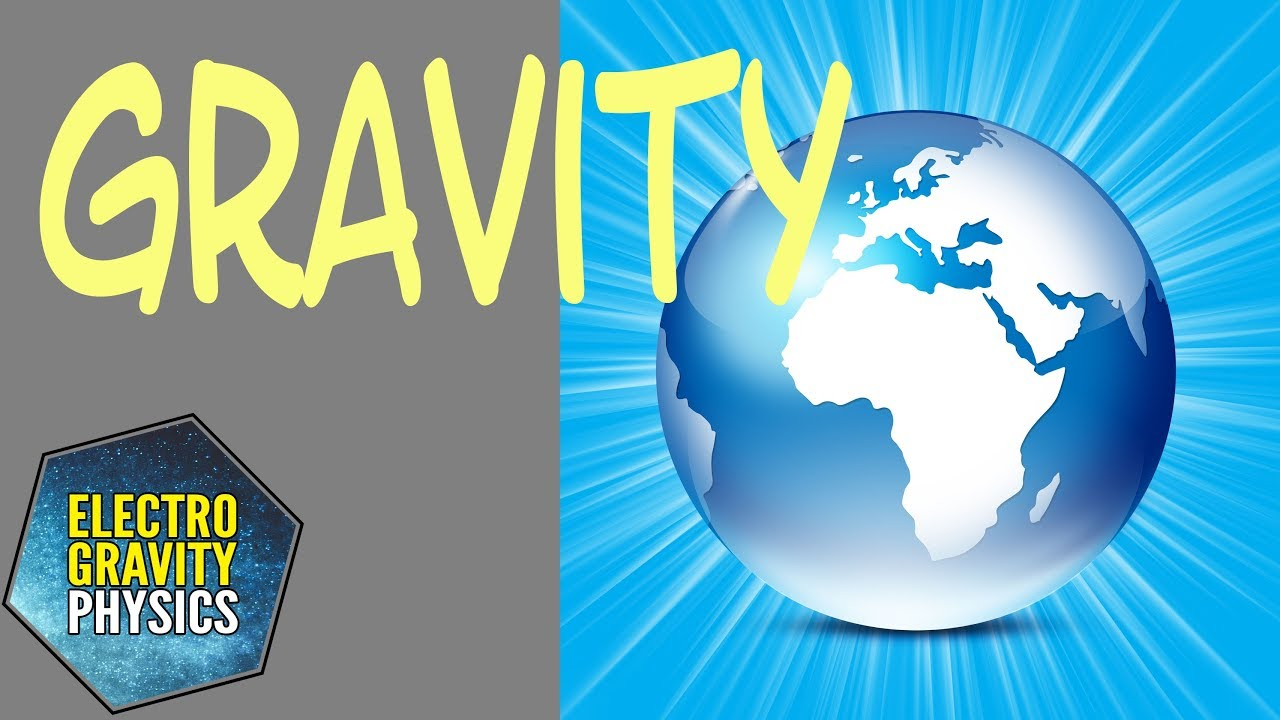# What is Gravity?

#### Definition of Gravity?

Gravity is a force of attraction between objects.

Conventional scientists do not know how to make Gravity.  They can calculate a rocket trajectory from Earth to the moon, but they do not know what causes gravity.  Scientists also do not know how to generate gravity artificially.

So, what is Gravity?  Einstein has talked about gravity as the curvature of time-space.  Other scientists talk about Gravity as made up quantum particles, called “gravitons”.  However, scientists have never found the graviton particle.

#### Time to consider an alternative explanation?

But as a result, what is gravity?  Perhaps there is a much simpler and alternative understanding of the cause of Gravity?

Nobel Laureate Prof. Richard Feynman said:  “Is it not possible that perhaps gravitation is due simply to the fact that we do not have the right coordinate system?”

Gravity might be explained as an electromagnetic side-effect.  (see the video below)   In other words, Gravity is not a fundamental force but simply a pseudo force.  As a result, Gravity is simply generated by ordinary orbital electrons.  This is due to motional magnetic fields of the Laplace Force in the Lorentz Equation.  This is seen as an electric field in the reference frame of other moving electrons of ordinary matter.#### An Electric Universe “Battery”?

The people that follow the “electric universe theory” have been looking for a way to explain the observed electric-like effects of the universe.  Now with a full understanding of the electro-gravity mathematics gravity can be understood and predicted.  Gravity is a high-frequency diverging Laplace force.

The Laplace force is caused by the motional electrons in all ordinary matter and is non-shieldable.   This effect is the ‘true’ cause of gravity, because the divergent electro-gravity field will “suck” all objects towards the center of the divergent electric field.

This also means that Gravity is not a fundamental force, because it is simply a side-effect of electromagnetics.

To read the full paper on why Gravity is an electromagnetic pseudo force, go to this link:

Free Fall of Elementary Particles

Let me know what you think and please leave your comments below.

-Nils Rognerud
San Francisco, CA, USA

If you enjoyed this article, click to get email updates… It’s Free